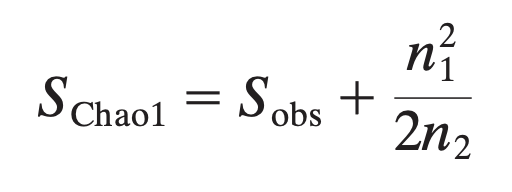# What are the different measures of calculating alpha-diversity supported in MicrobiomeAnalyst?

Alpha diversity is used to measure the diversity within a sample or an ecosystem. The two most commonly used alpha-diversity measurements are richness (count) and evenness (distribution). MicrobiomeAnalyst provides many metrics to calculate alpha diversity. Most commonly used ones are listed below:

• Richness: observed or estimated the number of unique species in the community (abundant or rare species are treated equally).

• Observed: the amount of unique OTUs found in each sample.

• ACE (abundance-based coverage estimators) and Chao1: also account for unobserved species based on low-abundance OTUs by adding a correction factor to the observed number of species. For instance, the Chao1 formula is given below (where Sobs is the observed species, n1 is the number of singletones, and n2 is the numbe rof doubletons• Evenness: These metrics account for both richness and abundance.

• Simpson and Shannon diversity measures are commonly used. Simpson diversity gives more weights to species with more frequency in a sample, while Shannon method gives more weights to rare species.
• Fisher’s alpha-diversity is widely used as a diversity index to compare among communities varying in number of individuals. It is scale independent and is defined implicitly by the formula S=a* ln*(1+n/a)* where S is number of taxa, n is number of individuals and a is the Fisher’s alpha